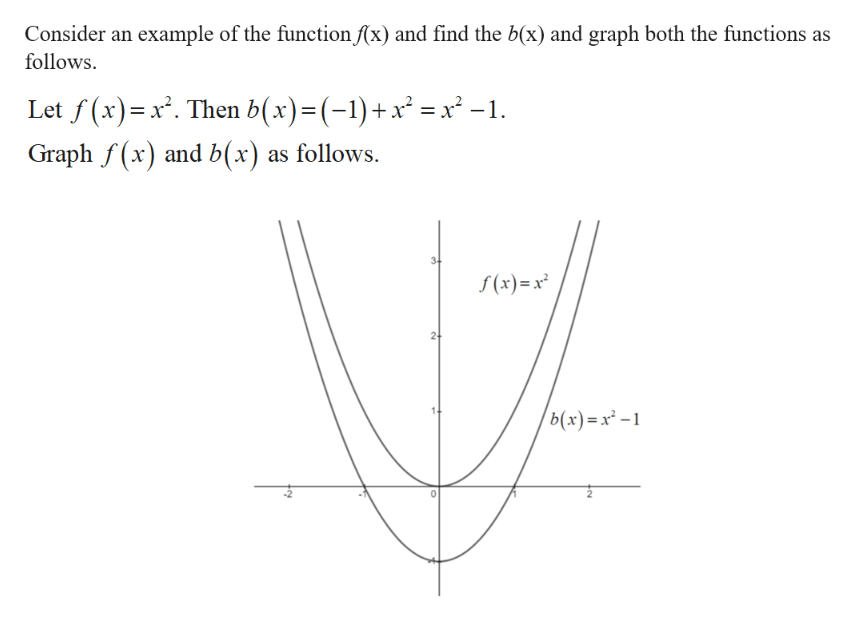# what does b(x) = (-1) + f(x) mean when shifting a graph

Question
1 views

what does b(x) = (-1) + f(x) mean when shifting a graph

check_circle

Step 1help_outlineImage TranscriptioncloseConsider an example of the function f(x) and find the b(x) and graph both the functions as follows Let f(x) x. Then b(x)=(-1)+x = x2 -1. Graphf(x and b(x) as follows. (x)x b(x)x- fullscreen

### Want to see the full answer?

See Solution

#### Want to see this answer and more?

Solutions are written by subject experts who are available 24/7. Questions are typically answered within 1 hour.*

See Solution
*Response times may vary by subject and question.
Tagged in

### Other